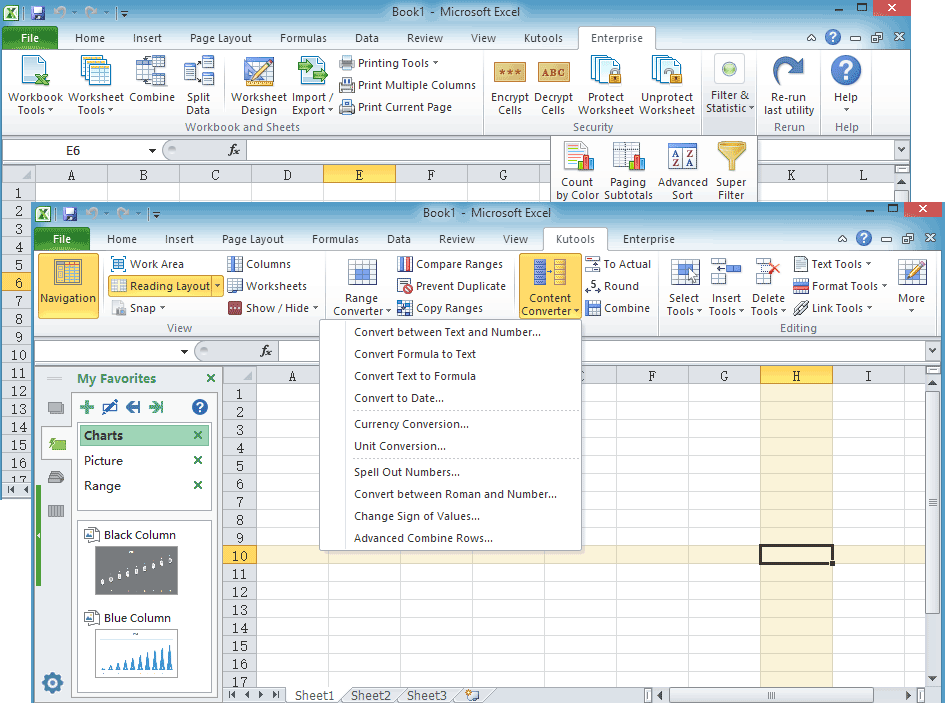## Quickly convert between various measurement units without using formula in Excel

### Kutools for Excel

Boosts Excel With 300+
Powerful Features

Without using formulas or functions, Kutools for Excel's Unit Conversion utility makes it easy to convert values between various measurement units, such as converting degrees to radian, converting minutes to hours, converting feet to inches and so on. With this utility, you can quickly convert between all kinds of measurement units without using formula in Excel.

Note: The Unit Conversion utility can convert between following measurements without using formula in Excel, including Angle measurements, Bits & Bytes measurements, Distance measurements, Energy measurements, Force measurements, Liquid measurements, Mass measurements, Surface measurements, Temperature measurements, Time measurements and Volume measurements.

#### Click Kutools >> Content >> Unit Conversion. See screenshot: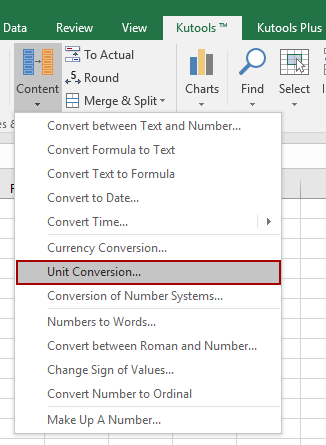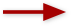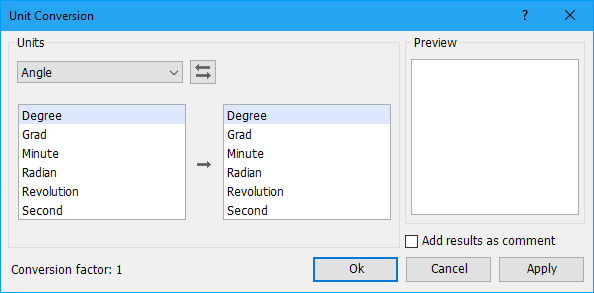#### If you are in a widescreen, please apply this utility by clicking Kutools > Conversion > Unit Conversion.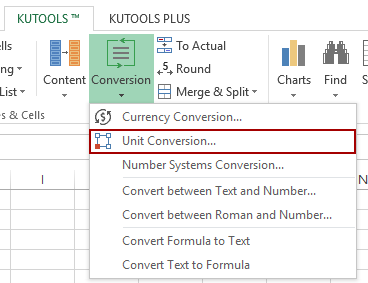#### Convert degrees to radians without using formula

Usually angles are measured in degrees, but sometimes you need to use radians to measure them. How do you convert degrees to radians or vise verse? With the Unit Conversion utility, you can quickly convert degrees to radians and other angle units without using formula or function.

1. Select a range of data that you want to convert to radians. Apply the utility by clicking Kutools > Content > Unit Conversion. (If you are in widescreen, click Kutools > Conversion > Unit Conversion.)

2. In the Unit Conversion dialog box, click the drop-down list under Units to choose Angle, and select Degree in left list box, then select Radian in right list box. At the same time, you can view the results from the Preview Pane. (Note: if you want to insert the results as comments of the original cells, please check Add results as comment.)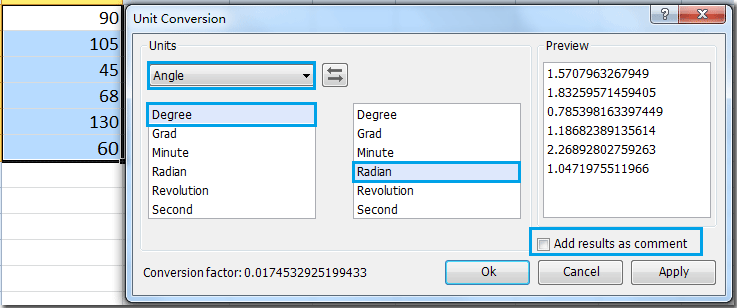3. Then click OK or Apply. You will get the following changes. Tip: Click OK button will close the dialog box and apply the operation; but click Apply button will only apply the operation without closing the dialog box. See screenshots: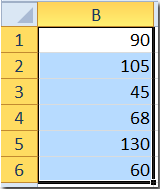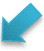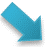Overwrite the original values Insert results as comment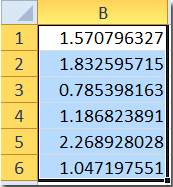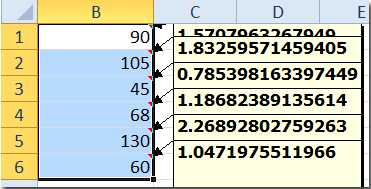Tip: with this Angle option, you can also do other conversions between this unit. Such as from Degree to Minute, from Radian to Second and so on.

#### Convert meters to feet, inches, miles or yards without using formula

As we all known, meter, yard or foot are widely used for length measurement in the word. So sometimes we need to do some conversions between them. With this Unit Conversion utility, you can quickly convert meters to feet, inches and other length units without using formula.

1. Select a range of data that you want to convert.

2. Go to the Unit Conversion dialog box, click the drop-down list under Units to choose Distance, and select Meter in left list box, then select Foot or other units that you need in right list box. Tip: If you want to insert the results as comments of the original cells, please check Add results as comment. See screenshot: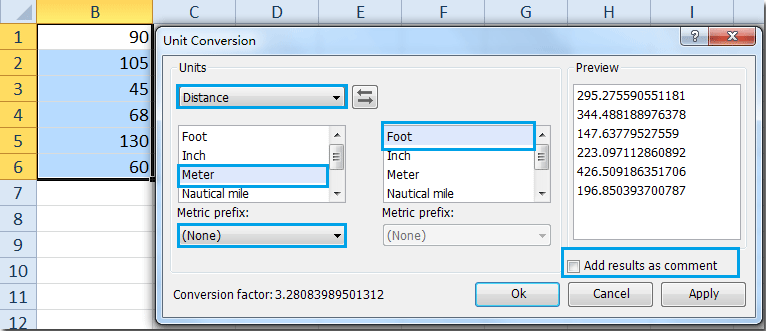3. Then click OK or Apply. You will get the following changes.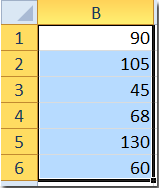Overwrite the original values Insert results as comment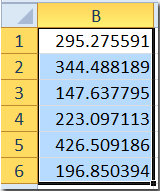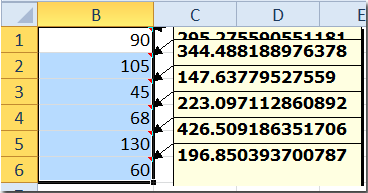Tips:

1. If you want to convert kilometers, centimeters, micrometers and so on to feet/inches/miles/yards, you can click the Metric prefix drop-down list to choose the units that you need.

2.with this option, you can also convert feet to inches, convert meters to yards and so on.

#### Convert Celsius to Fahrenheit or Kelvin without using formula

When you operate a worksheet, you are required to convert temperatures from Celsius to Fahrenheit or from Celsius to Kelvin or vise versa. If you have this utility Unit Conversion, you can easily convert between Celsius, Fahrenheit and Kelvin without formula.

1. Highlight the range of data that you want to change.

2. Go to the Unit Conversion dialog box, click the drop-down list under Units to choose Temperature, and select Celsius in left list box, then select Fahrenheit or Kelvin in right list box. Tip: if you want to insert the results as comments of the original cells, please check Add results as comment. See screenshot: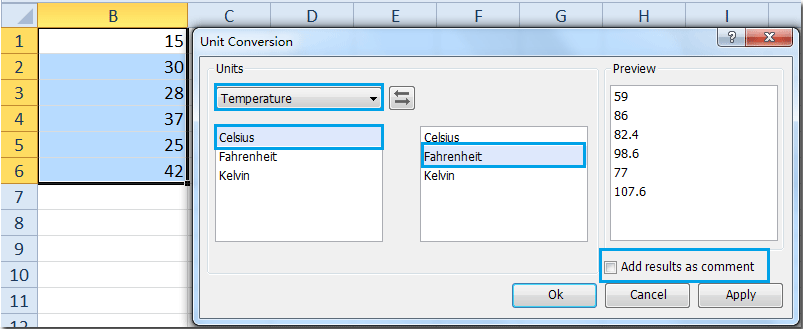3. Then click OK or Apply. The data of Celsius have been converted to Fahrenheit. You will get the following changes.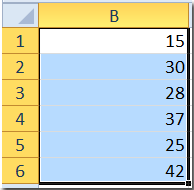Overwrite the original values Insert results as comment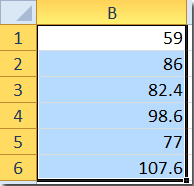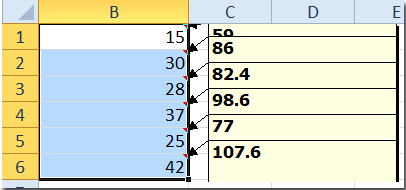Notes:

1. You can press Undo (Ctrl+Z) to restore this operation immediately.

2. Conversion factor shows the conversion ratio between two units.

3. This is only a few examples of this tool, with this utility you can do many other unit conversions.

#### Demo: Quickly convert between various measurement units without using formula in Excel

Productivity Tools Recommended
The following tools can greatly save your time and money, which one is right for you?
Office Tab: Using handy tabs in your Office, as the way of Chrome, Firefox and New Internet Explorer.
Kutools for Excel: More than 300 Advanced Functions for Excel 2021, 2019, 2016, 2013, 2010, 2007 and Office 365.

### Kutools for Excel

The functionality described above is just one of 300 powerful functions of Kutools for Excel.

Designed for Excel(Office) 2021, 2019, 2016, 2013, 2010, 2007 and Office 365. Free download and use for 30 days.